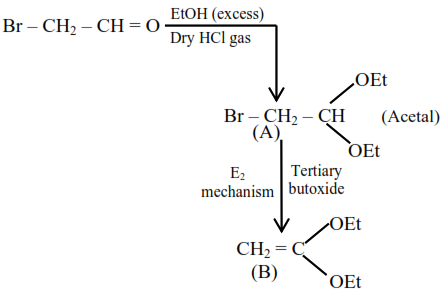# Solve thisQuestion: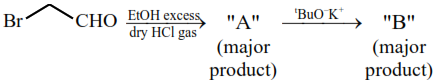$\left[\right.$ where $\left.\mathrm{Et} \Rightarrow-\mathrm{C}_{2} \mathrm{H}_{5}{ }^{\mathrm{t}} \mathrm{Bu} \Rightarrow\left(\mathrm{CH}_{3}\right)_{3} \mathrm{C}-\right]$

Consider the above reaction sequence, Product "A" and Product "B" formed respectively are :

1.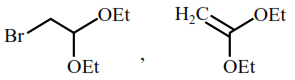2.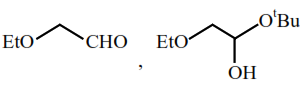3.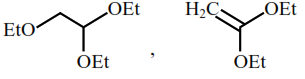4.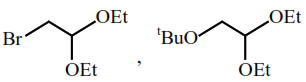Correct Option: 1

Solution: